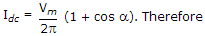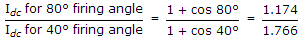# Electronics and Communication Engineering - Power Electronics - Discussion

### Discussion :: Power Electronics - Section 1 (Q.No.11)

11.

An SCR is triggered at 40° in the positive half cycle only. The average anode current is 50 A. If the firing angle is changed to 80°, the average anode current is likely to be

 [A]. 50 A [B]. 25 A [C]. less than 50 A but more than 25 A [D]. less than 25 A

Explanation:.

 Rupesh9292 said: (May 19, 2015) Please justify.

 Vamsi said: (Dec 5, 2016) Please explain it in clear.

 Hanu said: (Feb 9, 2018) Explain it clearly.

 Vivek Yadav said: (Feb 16, 2018) i for 80/i for 40=0.66, given i for 40 = 50, then i for 80=50*0.66=33 A so option c is correct.

 Jiaac said: (Sep 19, 2019) Since Vm is constant for the two cases, the ratio can be written as: (1+cos40°)/(1+cos80°) = 50A/X. Solve for X, and you get the average anode current of 33.23A for 80° firing angle. Since 33.23A is less than 50A but more than 25A, option C is correct.